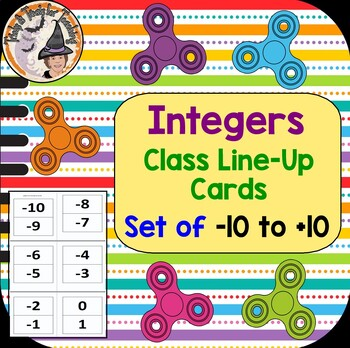# Integers Class Line Up Cards Ordering Real World Negatives Positives ActivitySubject
Resource Type
File Type
PDF (58 KB|10 pages)
Standards
\$2.25
• Product Description
• Standards

HELPFUL Integers-Class-Set of Line-Up-Cards are GREAT for a Real-World-Activity where you are physically having students line up to understand the ordering of Integers, using these great printables. You get 2 numbers per piece of copy paper. Just print and cut apart. Hand students one of the Integers (+10 thru -10 given) and have them line up in order. Great for comparing and ordering positive and negative integers. You'll enjoy using these helpful Integer cards year after year.

Be sure to L@@K at my other, 745+ TERRIFIC teaching resources!

~ ~ THANK YOU KINDLY ~ ~

Understand subtraction of rational numbers as adding the additive inverse, 𝘱 – 𝘲 = 𝘱 + (–𝘲). Show that the distance between two rational numbers on the number line is the absolute value of their difference, and apply this principle in real-world contexts.
Find and position integers and other rational numbers on a horizontal or vertical number line diagram; find and position pairs of integers and other rational numbers on a coordinate plane.
Recognize opposite signs of numbers as indicating locations on opposite sides of 0 on the number line; recognize that the opposite of the opposite of a number is the number itself, e.g., -(-3) = 3, and that 0 is its own opposite.
Understand a rational number as a point on the number line. Extend number line diagrams and coordinate axes familiar from previous grades to represent points on the line and in the plane with negative number coordinates.
Total Pages
10 pages